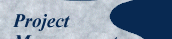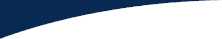Published here December 2004.

## Explanatory Preamble

To understand the following discussion you first have to know all the jargon, so here are the explanations for the alphabets soup.

The Earned Value Technique, loosely referred to as "EV" is a project management control tool defined as: "An objective measure of the value of work performed expressed in terms of the budget planned for the work." In other words, what you got for what you spent. The key acronyms are:

 EV = Earned Value = percent complete x corresponding budget BCWS = Budgeted Cost of Work Scheduled, now more properly known as the Planned Value (PV) ACWP = Actual Cost of Work Performed, i.e. the actual cost BCWP = Budgeted Cost of Work Performed, now more properly known as the Earned Value (EV) CV = Cost Variance = earned minus actual = BCWP-ACWP SV = Schedule Variance = earned minus budget = BCWP-BCWS BCWS-ACWP = Spending Variance = budget minus actual BAC = Budget At Completion EAC = Estimated (cost) At Completion EAC = variance at completion CPI = Cost Performance Index = BCWP/ACWP SPI = Schedule Performance Index = BCWP/BCWS Combined cost-schedule index = CPI x SPI

You can extrapolate performance to date to calculate the EAC as follows:

EAC = BAC/CPI = BACxACWP/BCWP

This calculation assumes, of course, that the performance experienced to date will continue unchanged to the end of the project. For a variety of reasons it never is and so this calculation is in fact of no practical value.

You can see the relationships of these various elements by going to http://www.maxwideman.com/issacons3/iac1343/sld006.htm